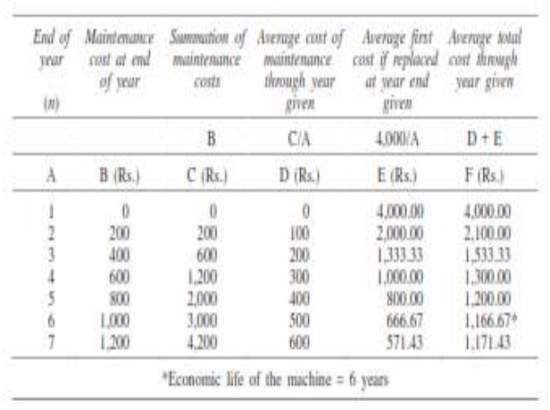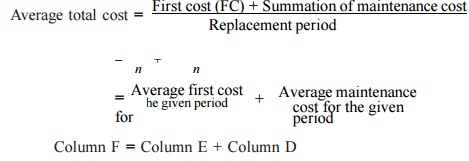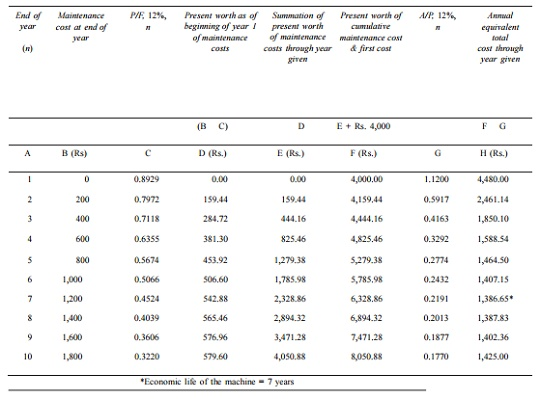Home | | Engineering Economics | Determination of Economic Life of an Asset

# Determination of Economic Life of an AssetAny asset will have the following cost components: ü Capital recovery cost (average first cost), computed from the first cost (purchase price) of the machine. ü Average operating and maintenance cost (O & M cost)

Determination of Economic Life of an Asset

Any asset will have the following cost components:

ü Capital recovery cost (average first cost), computed from the first cost (purchase price) of the machine.

ü Average operating and maintenance cost (O & M cost)

Total cost which is the sum of capital recovery cost (average first cost) and average maintenance cost.EXAMPLE

A firm is considering replacement of an equipment, whose first cost is Rs. 4,000 and the scrap value is negligible at the end of any year. Based on experience, it was found that the maintenance cost is zero during the first year and it increases by Rs. 200 every year thereafter.

(a)   When should the equipment be replaced if i = 0%?

(b)   When should the equipment be replaced if i = 12%?

(a)   When i = 0%. In this problem

(i)           First cost = Rs. 4,000

(ii)        Maintenance cost is Rs. 0 during the first year and it increases by Rs. 200 every year thereafter.

This is summarized in column B of TableColumn C summarizes the summation of maintenance costs for each replacement period. The value corresponding to any end of year in this column represents the total maintenance cost of using the equipment till the end of that particular year.

Average total cost = [ First cost (FC) + Summation of maintenance cost ] / Replacement periodColumn F = Column E + Column D

The value corresponding to any end of year (n) in Column F represents the average total cost of using the equipment till the end of that particular year.

For this problem, the average total cost decreases till the end of year 6 and then it increases. Therefore, the optimal replacement period is six years, i.e. economic life of the equipment is six years.

(b) When interest rate, i = 12%. When the interest rate is more than 0%, the steps to be taken for getting the economic life are summarized with reference to Table

Table Calculations to Determine Economic Life (First cost = Rs. 4,000, Interest = 12%)The steps are summarized now:

1. Discount the maintenance costs to the beginning of year 1.

Column D = Column B

1/ (1 + i)n

= Column B    (P/F, i, n) = Column B    Column C.

2. Find the summation of present worth of maintenance costs through the year given (Column E = Column D).

3. Find Column F by adding the first cost of Rs. 4,000 to Column E.

4. Find the annual equivalent total cost through the years given.

Column H = Column F       i(1 + i)n                 / (1 + i)n      − 1

= Column F     (A/P, 12%, n) = Column F    Column G

5. Identify the end of year for which the annual equivalent total cost is minimum.

For this problem, the annual equivalent total cost is minimum at the end of year 7. Therefore, the economic life of the equipment is seven years.

Study Material, Lecturing Notes, Assignment, Reference, Wiki description explanation, brief detail
Mechanical : Engineering Economics & Cost Analysis : Replacement And Maintenance Analysis : Determination of Economic Life of an Asset |